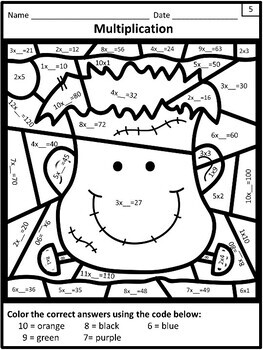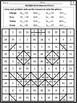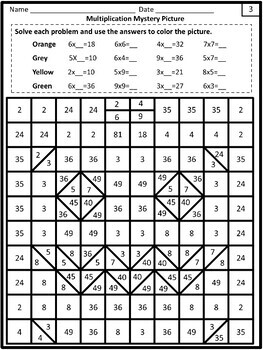# Multiplication Worksheets for HalloweenSubject
Resource Type
File Type

PDF

(5 MB|17 with cover, answer keys and credits)
Product Rating
Standards
• Product Description
• StandardsNEW

Do your students need more practice with basic multiplication facts? The 10 worksheets in this resource will have them engaged and mastering their facts and will keep them engaged on the days leading up to Halloween!

You will find 10 worksheets that will include color by code, mystery pictures, picture puzzles and cute segmented circle designs. All 10 worksheets have answer keys.

Fluently multiply and divide within 100, using strategies such as the relationship between multiplication and division (e.g., knowing that 8 × 5 = 40, one knows 40 ÷ 5 = 8) or properties of operations. By the end of Grade 3, know from memory all products of two one-digit numbers.
Apply properties of operations as strategies to multiply and divide. Examples: If 6 × 4 = 24 is known, then 4 × 6 = 24 is also known. (Commutative property of multiplication.) 3 × 5 × 2 can be found by 3 × 5 = 15, then 15 × 2 = 30, or by 5 × 2 = 10, then 3 × 10 = 30. (Associative property of multiplication.) Knowing that 8 × 5 = 40 and 8 × 2 = 16, one can find 8 × 7 as 8 × (5 + 2) = (8 × 5) + (8 × 2) = 40 + 16 = 56. (Distributive property.)
Total Pages
17 with cover, answer keys and credits
Included
Teaching Duration
N/A
Report this Resource to TpT
Reported resources will be reviewed by our team. Report this resource to let us know if this resource violates TpT’s content guidelines.